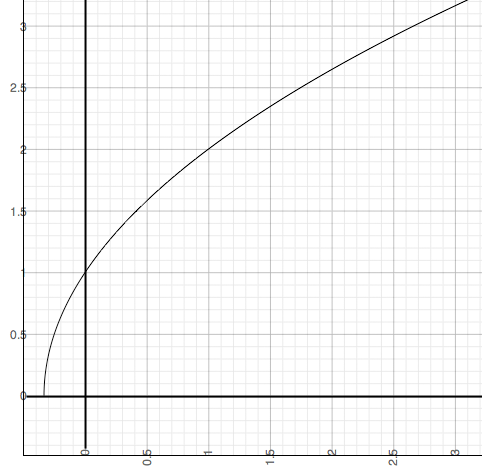SEARCH HOMEMath Central Quandaries & QueriesQuestion from Warren, a teacher: What are the conditions of the numerical coefficients of the standard form Ax+By=C in Linear Function? How will you transform y=square root of 3 x + 1 in standard form Ax+By=C?Warren,

A linear function is one that can be written in the form Ax + By = C where A, B and C are any real numbers. The term linear comes from the fact that its graph is a straight line. The graph of y = √(3x + 1) is not a straight liney = √(3x + 1)

and hence the expression y = √(3x + 1) can not be written in the standard form Ax + By = C where A, B and C are real numbers.

PennyMath Central is supported by the University of Regina and The Pacific Institute for the Mathematical Sciences.